Math Concepts
Finding a Perfect Square
28.5k views

### June 12, 2020

After the basic mathematical operations are taught to a student, he/she is exposed to certain higher-level mathematical operations. Finding the square root of a number is one of them. The square root of a number (let’s say 25) refers to that number which when multiplied by itself will give the result as the given number (i.e. 25). In our case, the number is 5. We see that 5, when multiplied by itself (i.e. 5), will result in 25. Therefore, 25 is known as the square of 5, and 5 is known as the square root of 25.

Note:

(1) Multiplying two negative numbers will also result in a positive number. Therefore, (-5)*(-5) is also equal to 25. So, -5 is also a square root of 25. But, in general, we assume the square roots of a positive number to be a positive number.

(2) The square root of 1 is always 1.

We represent the square root of a number by a radical sign (√). Example: √36 = 6 (because 6 x 6 = 36)

Note:

(3) Finding the square root of a negative number (i.e. when the negative sign is inside the radical sign i.e. √-36) is a bit complex and is out of context for this article.

(4) if the minus sign is given outside the radical sign (i.e. -√36), then the answer will always be -6.

There are two types of numbers:

• Numbers which are a perfect square: Product of an integer with itself. For example, 9*9 = 81.

• Numbers which are an imperfect square: Multiplying any number with itself. For example, 2.236*2.236 = 5.

Finding the square root of an imperfect square is a little more difficult than finding the square root of a perfect square number. We will be covering both the topics in this particular article. But before that, we have to understand what square roots are used for.

For example, Rhea is looking for an apartment in Delhi. She is looking for a 2 bedroom apartment where she can live with her sister. A few phone calls later, she has shortlisted two places after seeing their pictures online. One is 1600 square feet with a balcony and the other is 2500 square feet with two balconies. Now, she can easily gauge the size of the first apartment as 40 feet by 40 feet. The second apartment would be 50 feet by 50 feet. This was just an example. Square roots are used for multiple purposes like these.

Now coming to our main topic, i.e., how to find the square root of a number?

## (A) Perfect Square Numbers:-

Before finding the square roots of a number, we have to know the squares of the first 9 natural numbers.

1*1 = 1

2*2 = 4

3*3 = 9

4*4 = 16

5*5 = 25

6*6 = 36

7*7 = 49

8*8 = 64

9*9 = 81

From the above chart, we can conclude the following:

• A perfect square cannot have 2, 3, 7, or 8 in the unit’s digit.

• If a perfect square ends with a 1, then the unit’s digit of its square root has to be either 1 or 9.

• If a perfect square ends with a 4, then the unit’s digit of its square root has to be either 2 or 8.

• If a perfect square ends with a 5, then the unit’s digit of its square root is definitely 5.

• If a perfect square ends with a 6, then the unit’s digit of its square root has to be either 4 or 6.

• If a perfect square ends with a 9, then the unit’s digit of its square root has to be either 3 or 7.

Note: Firstly, we have to find out the number of digits in the answer.

• The square root of a number with an odd number of digits (for example, 24563 has 5 digits i.e. odd) will have (n+1)/2 digits in the answer (in our example, (5+1)/2 = 3 digits in the answer).

• The square root of a number with an even number of digits (for example, 2456 has 4 digits i.e. even) will have n/2 digits in the answer (in our example, 4/2 = 2 digits in the answer).

We will understand the further steps with the help of an example. Let us say, we have to find out the square root of 2116.

(1) The number of digits in our answer will be 4/2 = 2

(2) We will divide 2116 into 2 groups. 21 and 16.

Note: If we had taken a 3-digit number like 121, we would have considered 01 and 21. Similarly, if we would have taken a five-digit number like 11025, we would have considered 110 and 25. This shows that the second pair must always have only the last two digits.

(3) The nearest square to the first pair i.e. 21 is 16 and 25. We will always take the smaller one. The square root of 16 is 4. So our first digit of the final answer is 4.

(4) Now let's come to the second pair i.e. 16. Check the unit’s digit. In our case, it is 6 which means that the square root is either 4 or 6 as mentioned in the description. Since the first digit of the square root is 4 and we know that it’s a two-digit number, the square root of 2116 could either be 44 or 46. This lies between 40 and 50.

(5) Next, we have to square 40 and 50, we get 1600 and 2500 respectively.

(6) Figure out which of these numbers is closer to the given question. In our case, 2116 is closer to 50. Therefore, the square root of 2116 is 46.

A regular practice can make you perform these steps in a quick manner.

## (B) Imperfect Square Numbers:-

Finding out the square roots of imperfect numbers follows a different approach i.e. division method. Let us understand, how to find a square root by division method? (Please note, that the division method can also be applied to the perfect square numbers).

Let us say, we have to find out the square root of 180.

Step 1: Place a bar over every pair of the digit of the number starting from that in the unit’s place (rightmost side). So we will have two pairs in our case, i.e. 1 and 80. (For example, if we had 16673 as our number then we would have 3 pairs i.e. 1, 66 and 73)

Step 2: We divide the left most number by the largest number whose square is less than or equal to the number in the leftmost pair.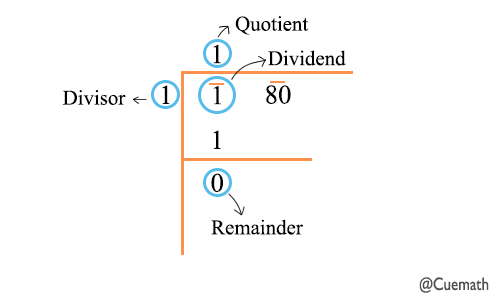Step 3: Bring down the number under the next bar to the right of the remainder. Add the last digit of the quotient to the divisor. To the right of the obtained sum, find a suitable number which together with the result of the sum forms a new divisor for the new dividend that is carried down.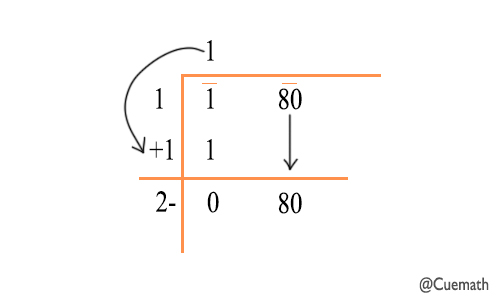Step 4: The new number in the quotient will have the same number as selected in the divisor. The condition is the same as being either less or equal to that of the dividend.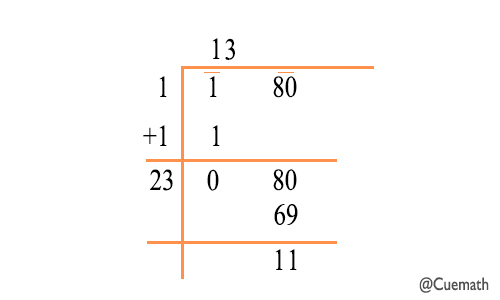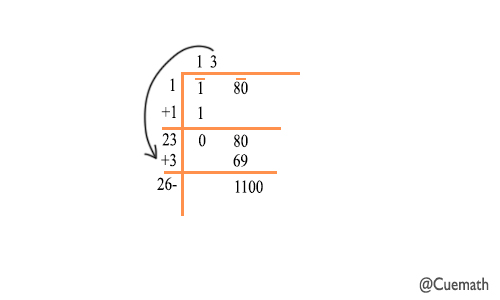Step 5: Now, we will continue this process further using a decimal point and adding zeros in pairs to the remainder.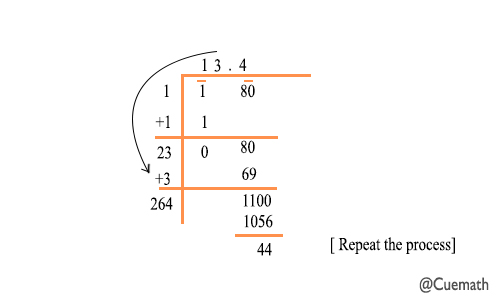Step 6: Our answer as shown in the quotient will be something equal to 13.4xxx. The quotient thus obtained will be the square root of the number.

There is yet another method to find the square root of a number. This is known as the Prime Factorisation Method.

Step 1: As the name suggests in this method we need to find the prime factors of the given number.

Step 2: Make pairs of prime factors.

Step 3: Take the product of one factor from each pair.

Let us find the square root of 441.

The prime factorization of 441 is 3 × 3 × 7 × 7.

Make the pair and select one factor from each pair that gives 3 × 7 = 21.

So, the square root of 441 = √441 = 21.

Similarly, let us find the square root of 180.

The prime factorization of 180 is 180 = 2 × 2 × 3 × 3 × 5.

While making the pair we say that five is not a pair.

Therefore 180 is not a perfect square and our answer will be √180 = 2 × 3 × √5 = 6√5.

Finding the square root of decimal numbers is very similar to finding the square roots of integers. The only difference is in placing a bar over the numbers before and after the decimal point. We place the bar over every pair of the number starting from the unit’s place (before the decimal point). And we place the bar over every pair of the number starting from the beginning of the number after the decimal point. For example, if we have to find the square root of the number 121.782, then our pairs will be 1, 21, 78, and 2. We have to now follow the same process of division method.

Some of the other frequently asked questions and examples are as follows:-

1. How to find the square root of 2?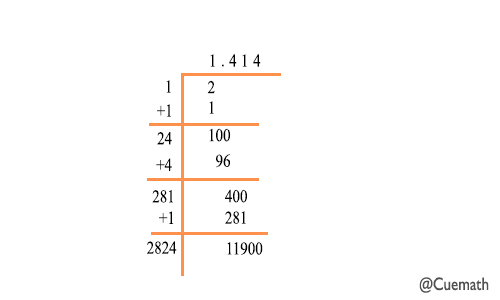1. How to find the square root of 6?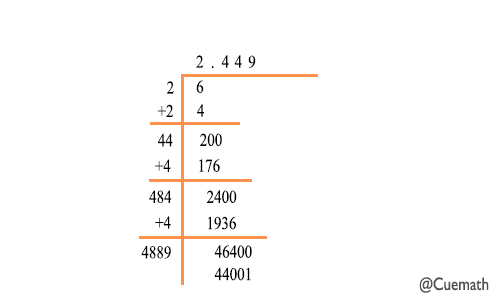1. How to find the square root of 5?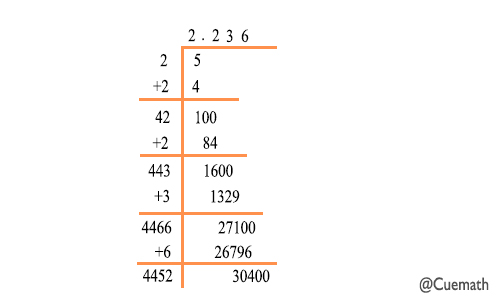1. How to find the square root of 35?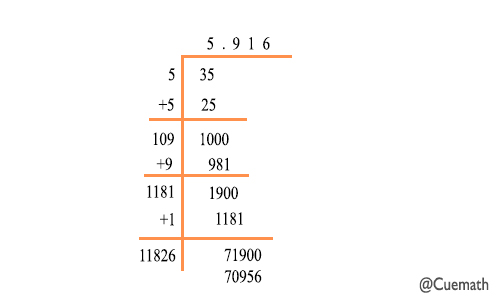Cuemath comes with regular such blog articles to clear different concepts of students. We ensure that we explain each and every step in the most detailed manner possible. We also make sure that we provide as many examples as possible while explaining the concepts to our students. Wherever possible, we also try to inculcate practical examples while providing explanations. Through our maths Online Classes we provide regular support to our students at every phase possible. Through our maths gym app, which is available for both iOS and Android platforms, we try to build the mental abilities and skills of our students. We guarantee our support at every step and we also provide due feedback for every task. Join Cuemath for a bright future.

Happy Studying!!

Best of Luck!!

Related Articles